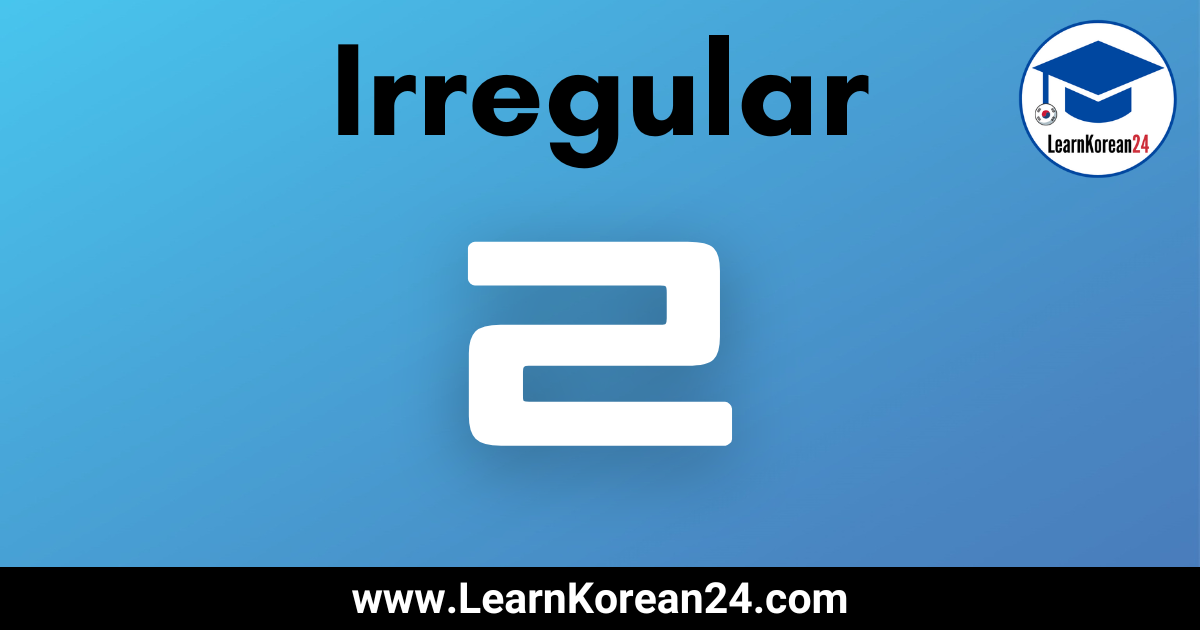# Irregular Korean Verbs With ㄹ (ㄹ 불규칙)In this lesson, you will learn about ㄹ irregular verbs (ㄹ 불규칙). In Korean, all verbs and adjectives which end in ㄹ are irregular. This means that verbs and adjectives which end in ㄹ are conjugated differently compared to regular verbs and adjectives.

Below you will find a ㄹ Irregular Verbs List and you will learn how to conjugate ㄹ irregular verbs.

## ㄹ Irregular (ㄹ 불규칙)

As was mentioned above, any verb or adjective which ends in is irregular. Here is a list of irregular verbs and adjectives.

• 길다 = to be long
• 놀다 = to play
• 멀다 = to be far
• 알다 = to know
• 열다 = to open
• 울다 = to cry
• 팔다 = to sell
• 만들다 = to make
• 힘들다 = to be hard/difficult
• 달다 = to be sweet
• 불다 = to blow
• 걸다 = to hang
• 밀다 = to push
• 들다 = to hold
• 풀다 = to untie/undo
• 늘다 = to grow
• 갈다 = to replace
• 물다 = to bite
• 거칠다 = to be rough
• 기울다 = to tilt

## How To Conjugate Irregular ㄹ Verbs (ㄹ 불규칙)

Of the seven different types of irregular verbs in Korean, ㄹ irregular verbs are one of the most difficult to learn. This is because not only are they conjugated differently to regular verbs, the correct conjugation is unlike the other irregular verb forms.

Here are the conjugation rules to follow when conjugating ㄹ irregular verbs and adjectives. The conjugation rule to follow is determined by the ending you want to attach to the verb / adjective.

### Rule 1: Endings Starting With ㄴ,ㅂ,ㅅ

To conjugate ㄹ irregular verbs with endings starting with ㄴ,ㅂ,or ㅅ, ㄹ is dropped from the verb stem.

For example, 살다 (to live) + the ending 네요 becomes 사네요. This is because ㄹ is dropped from the verb stem before adding the ending. Here are some more examples of endings which follow this conjugation rule:

### Rule 2: Endings Starting With 으 or 스

This next conjugation rule is where things get more complicated.

When conjugating ㄹ irregular verbs with endings starting with or , the first thing you must do is drop 으 / 스 from the ending. Here are some examples of endings which start with 으 or 스:

After dropping 으 / 스 from the ending, the next thing you must do is decide whether or not to drop ㄹ from the verb stem. Take a look at these two examples:

1. 살다 + (으)니까 = 사니까
2. 살다 + (으)면 = 살면

As you can see, in both these examples, 으 is dropped from the ending. However, ㄹ is only dropped from the verb stem in the 1st example (사니까).

This is because, after dropping the from (으)니까, you’re left with 니까. As you learned in the first conjugation rule above, when you are conjugating irregular ㄹ verbs with endings that start with ,, or , ㄹ is dropped from the verb stem.

In the 2nd example (살면), ㄹ is not dropped from the verb stem. This is because after dropping 으 from the ending (으)면 you are left with 면. 면 does not start with ㄴ, ㅂ, or ㅅ, and so you do not need to drop the ㄹ from the verb stem.

Take a look at these examples and notice which ones have dropped the ㄹ from the verb stem.

As you can see, in the first 3 examples in the table above, 으 is dropped from the ending AND ㄹ is dropped from the verb stem. In the last 2 examples, only 으 is dropped from the ending.

As was mentioned above, this conjugation rule can be a little difficult to learn at first, but overtime as you learn and speak more Korean, these conjugations will become second nature.

When conjugating irregular ㄹ verbs with endings which start with 으 or 스, the first thing you must do is drop 으 / 스 from the ending. Next, you must decide whether or not to drop ㄹ from the verb stem. To do this, check if after removing 으 / 스 from the ending you are left with an ending starting with ㄴ, ㅂ, or ㅅ. If you are, then drop ㄹ from the verb stem. If not, then don’t drop the ㄹ from the verb stem.

### Rule 3: Endings Starting With (으)ㄹ

This next conjugation rule is quite simple. When you want to conjugate a ㄹ irregular verb with an ending which starts with (으)ㄹ, drop (으)ㄹ from the ending.

For example, 만들다 (to make) + (으)ㄹ 거예요 (future tense ending) = 만들 거예요.

Here are some more examples which follow this rule:

### Rule 4: All Other Endings

When conjugating an irregular ㄹ verb with an ending which is different to the ones mentioned above, then there is no special conjugation rule. They are conjugated just the same as regular verbs and adjectives.

For example, 알다 (to know) + 아요/어요 (present tense) = 알아요. In this example, just like regular verbs, the verb stem does not change.

Here are some more examples which follow this conjugation rule:

## ㄹ Irregular Example Sentences

Here are some useful ㄹ irregular example sentences:

• 어디에 사세요? (살다) = Where do you live?
• 우리 집은 직장에서 멉니다. (멀다) = My house is far from my workplace.
• 더워요? 창문을 좀 열까요? (열다) = Are you hot? Shall I open the window?
• 우리 내일 같이 놀까요? (놀다) = Shall we hang out tomorrow?
• 외국 생활은 힘들지만 재미있어요. (힘들다) = Living overseas is hard, but it is fun.

## More Korean Irregular Verb Lessons

There are seven types of irregular verbs in Korean. For more lessons on Korean Irregular verbs, click on the links below.